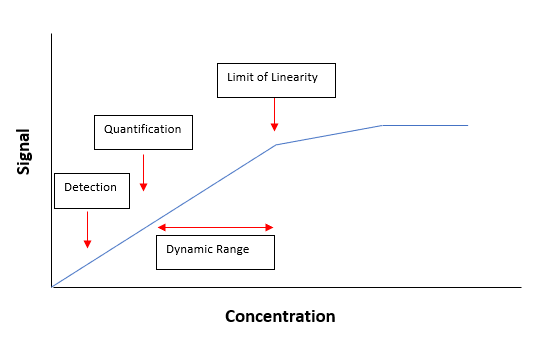# What are the definitions and differences of common Detection and Quantification Limits?

## Dokument-ID

Dokument-ID TE973

## Veröffentlichungsdatum

Veröffentlichungsdatum 04.01.2022
Frage
What are the definitions and differences of common Detection and Quantification Limits?
Zusammenfassung
Explanation of differences between Estimated Detection Limits (EDL), Method Detection Limits (MDL), and Practical Quantification Limits (PQL)
Antwort

Introduction:

Ranges for chemical measurements have limits. The lower limits are important because they identify whether a measurement is different from zero and if it can be quantified.

There are two distinct classes of reporting limits: detection limits and quantification limits. Detection limits refer to a minimum concentration of an analyte that can be detected. Quantification limits refer to a minimum concentration of an analyte that can be measured within specified limits of precision and accuracy. The following picture illustrates the relationship between detection and quantification (the example given below is for a linear calibration curve which has a limit of linearity):There are multiple kinds of detection and quantification limits. The most common ones used by Hach are defined below.

Please note that other detection and quantification limits exist. Definitions and procedures for determining these limits may vary. Customers should speak with their regulators to determine what limits are appropriate for them.

Estimated Detection Limit (EDL):

The lowest value stated in most Hach procedures is the EDL, and is generally based on the Sensitivity value. The EDL is a useful starting point when a MDL is to be found. It is also useful for comparing methods. The values are typically set via precision studies during the method development process, and/or during the instrument calibration. These tests are performed under ideal conditions, and current methods state that users may obtain different results.

The following article contains additional information on the Sensitivity Value:

What is the sensitivity of a Hach method?

Method Detection Limit (MDL):

The USEPA defines the MDL as the minimum concentration that can be found with a 99% level of confidence that the true concentration is higher than zero. A measurement below this MDL is highly suspect.

The MDL is not fixed. It is different for each reagent lot, instrument, analyst, sample type, etc. Therefore, a published MDL may be a useful guide, but is only accurate for a specific set of circumstances. Each analyst should find a more accurate MDL for each specific sample matrix with the same equipment, reagents and standards that will routinely be used for measurements. Since the MDL is different from analyst to analyst, it is important that the MDL be found under actual operating conditions.

The following procedure to find the MDL  is based on replicate analyses at a concentration one to five times the EDL. The MDL value is calculated from the standard deviation of the replicate study results multiplied by the appropriate t value for a 99% confidence interval. For this definition, the MDL does not account for variation in sample composition and can only be achieved under ideal conditions.

1. Make an estimate of the detection limit. Use the EDL or sensitivity value stated in the Method Performance section fo the analysis procedure.
2. Prepare a laboratory standard of the analyte, one to five times the EDL, in deionized (DI) water that is free of the analyte.
3. Make an analysis of at least seven portions of the laboratory standard and record each result.
4. Calculate the average and the standard deviation(s) of the results.
5. Calculate the MDL with the approate t value and the standard deviation value:  MDL = t x s

The following Table gives common t values:

 Number of Test Portions t Value 7 3.143 8 2.998 9 2.896 10 2.821

Practical Quantification Limit (PQL):

Sometimes referred to as the ML, the PQL is the lowest concentration that can be reliably achieved within specified limits of precision and accuracy during routine laboratory operating conditions. It is often defined as the lowest quantification value at 95% confidence. The PQL is usually calculated based on the MDL.

Anhänge
Anhänge

## Verwandte Antworten

### Hat dir diese Antwort geholfen?

Wird gesendet...
Vielen Dank für Ihr Feedback.
Beim Senden ist ein Fehler aufgetreten. Versuchen Sie es erneut.